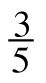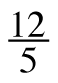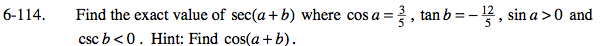### Home > PC > Chapter 6 > Lesson 6.3.1 > Problem6-114

6-114.

Find the exact value of sec(a + b) where cos a =, tan b = −, sin a > 0 and csc b < 0. Hint: Find cos(a + b). Homework Help ✎Sketch two triangles for this situation. One for angle a and one for angle b.